# Magnetic Pendulum

A chaotic situation arises in the experiment of swinging a magnetic pendulum over a plane containing two or more attractive magnets: which magnet the pendulum ultimately rests over is highly dependent on the starting position and velocity of the pendulum, so that small variations in initial conditions lead to different results.

The situation with three magnets can be modeled with the following pair of differential equations: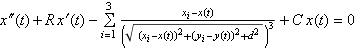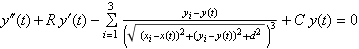where the xi and yi are the coordinates of the positions of the three magnets; x(t) and y(t) are the positions of the pendulum at time t; d is the distance between the pendulum and the plane containing the magnets; and R and C are friction and spring (self-restoring force) parameters.

The situation can be modeled easily in Mathematica using `NDSolve` (so I won’t type in the code); in each of the following graphs, the pendulum is given a slightly different starting position (with identical initial velocity, etc.), and in each picture the pendulum ends up over a different magnet.

x(0) = -0.95, y(0) = 0.09: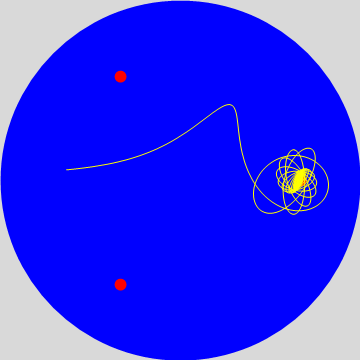x(0) = -0.95, y(0) = 0.095: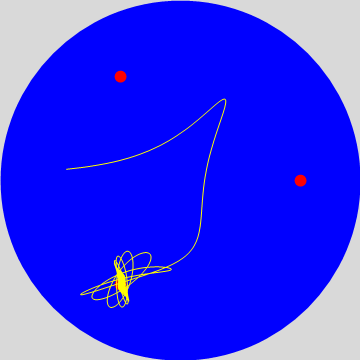x(0) = -0.95, y(0) = 0.1: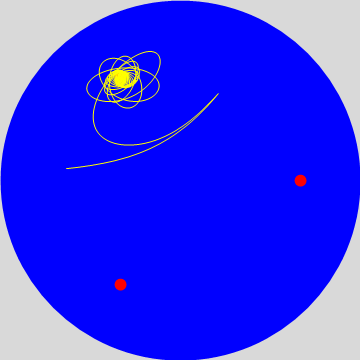(Sorry about the repulsive coloring scheme; I don’t know what I was thinking. The red dots represent the three magnets, and the yellow squiggle is the path of the pendulum. If such things interest you, I arbitrarily chose R = 0.2, C = 0.5, d = 0.25, and x′(0) = y′(0) = 0. It might even interest you to know that the basins of attraction formed by the magnetic-pendulum model are very similar in character to the basins of attraction formed by using Newton’s method to find complex roots to an equation.)

Equations pinched from Peitgen et al, Chaos and Fractals, Springer-Verlag, 1992.